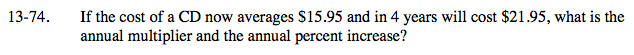### Home > A2C > Chapter Ch13 > Lesson 13.1.4 > Problem13-74

13-74.

If the cost of a CD now averages $15.95 and in 4 years will cost$21.95, what is the annual multiplier and the annual percent increase? Homework Help ✎15.95r4 = 21.95

r = 1.083

What percent increase is this?